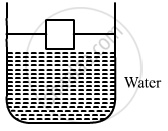Department of Pre-University Education, KarnatakaPUC Karnataka Science Class 11
Advertisement Remove all ads

# A Cubical Box is to Be Constructed with Iron Sheets 1 Mm in Thickness. What Can Be the Minimum Value of the External Edge So that the Cube Does Not Sink in Water? Density of Iron = 8000 Kg M−3 - Physics

Sum

A cubical box is to be constructed with iron sheets 1 mm in thickness. What can be the minimum value of the external edge so that the cube does not sink in water? Density of iron = 8000 kg/m3 and density of water = 1000 kg/m3.

Advertisement Remove all ads

#### Solution

Given:
Density of iron, ρI = 8000 kg/m3 = 8 gm/u
Density of water, ρw = 1000 kg/m3 = 1 gm/u
Let x be the external edge of iron.
According to Archemedes' principle,
Weight displaced = Upward thrust
∴ w = uFor the given condition, we have:
Weight of the box = Buoyant force
⇒ V1 ρIg = vρwg

⇒ (x2 × (0.1) × 6) × 8 = x3 × 1            [Volume of iron = v1 = 6  times the volume of each sheet]
⇒ x = 4.8 cm

Is there an error in this question or solution?
Advertisement Remove all ads

#### APPEARS IN

HC Verma Class 11, 12 Concepts of Physics 1
Chapter 13 Fluid Mechanics
Q 15 | Page 274
Advertisement Remove all ads

#### Video TutorialsVIEW ALL 

Advertisement Remove all ads
Share
Notifications

View all notifications

Forgot password?